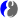# DMelt:DSP/1 Fast Fourier Transforms

Limitted access. First login to DataMelt if you are a full DataMelt member. Then login to HandWiki as a user.

# Fast Fourier transforms

A Fast Fourier transform (FFT) is an algorithm that samples a signal over a period of time (or space) and divides it into its frequency components. There are several packages dealing with the FFT problem. We will discuss the fastest one, which uses many threads and the multicore computer architecture. It is based on the ParallelColt as implemented by Piotr Wendykier.

In this example we will useDComplexFactory2D factory to create a random dense matrix (DenseDComplexMatrix2D) and perform FCC.

# Discrete Cosine Transform (DCT)

A Discrete cosine transform discrete cosine transform (DCT) expresses a finite sequence of data points in terms of a sum of cosine functions oscillating at different frequencies. This example is based on the realDenseDoubleMatrix2D matrix.

You can also use 3D matrix methods, seeDenseDoubleMatrix3D

# Discrete Fourier transform (DFT)Discrete Fourier transform converts a finite sequence of equally-spaced samples of a function into a same-length sequence of equally-spaced samples of the discrete-time Fourier transform. This is 1D DFT transform of a complex matrix 1000x1000 using several processing cores: